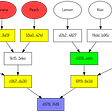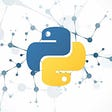# Numerical analysis

`class DumbIntegrator:    """ The dumbest possible numerical integrator """    def __init__(self, m0 = 0):        self.m0 = m0        self.y0 = None    def calculate(self, dt, y):        y0 = self.y0 if self.y0 else y        new_area = dt*((y0 + y)/2)        total_area = self.m0 + new_area        # update state        self.y0 = y        self.m0 = total_area        return total_areaclass DumbDifferentiator:    """ The dumbest possible numerical derivator """    def __init__(self):        self.y0 = None    def calculate(self, dt, y):        y0 = self.y0 if self.y0 else y        rate = (y - y0)/dt        # update state        self.y0 = y        return rate`

# PID regulator

`from .base import BaseModelfrom ..utils.numerical_analysis import DumbDifferentiator, DumbIntegratorclass PID(BaseModel):    """ A class used to model a dumb PID regulator """    def __init__(self, dt, Kp, Ti, Td, error = 0):        # constants        self.dt = dt        self.Kp = [Kp]        self.Ti = [Ti]        self.Td = [Td]        # state variable        self.error = [error]        # inputs        self.PV = [None]        self.SP = [None]        # other        self.I = DumbIntegrator()        self.D = DumbDifferentiator()        self.PG =         self.IG =         self.DG =         # output        self.MV =     def calculate(self, SP, PV, FWD):        """ Calculates the next value and adds it to the memory """        Kp = self.Kp[-1]        Ti = self.Ti[-1]        Td = self.Td[-1]        # error        error = SP - PV        # gains        PG = Kp*error        IG = self.I.calculate(self.dt, error)*Kp/Ti        DG = self.D.calculate(self.dt, error)*Kp*Td        # output        MV = PG + IG + DG + FWD        # update attributes        self.update_attributes(**{            "error": error,            "PG": PG,            "IG": IG,            "DG": DG,            "MV": MV        })        return MV`

# What’s next

--

--

--

## More from Fábio Molinar

Back-End Web developer, Industrial Automation Engineer, Husband & Father.

Love podcasts or audiobooks? Learn on the go with our new app.

## Exploring Sign Language Recognition techniques with Machine Learning## Long/Short Equity Strategies And Machine Learning## “Autonomous vehicle” Science-Research, October 2021, Week 2 — summary from Arxiv and Springer…## Understanding sparse Merkle multiproofs## Political sentiment of British media in 2019## Catalyst 101 — Accelerated PyTorch## Getting Started with Python & Machine Learning from Scratch## The Motivation to Switch to Convolutional Neural Networks.## Fábio Molinar

Back-End Web developer, Industrial Automation Engineer, Husband & Father.

## Building A Metronome In Python## Do Left Handed People Really Die Young — Datacamp datasets## Integration with SymPy or SciPy## How much land would it take to run the Netherlands on solar energy?